### 关于f(x) 和 f(-x) 表示什么数学含义：

 标签(TAG)： 关于f(x) 和 f(-x) 表示什么数学含义： 关于f(x) 和 f(-x) 表示什么数学含义： 1、当一个函数f(x)的定义域为(－∞，＋∞)时，当自变量x取两个相反数x、-x时，对应的函数值分别为f(x)、f(-x)； 1）f(x)=f(-x)，f(x)为偶函数； 2）f(x)=-f(-x)，f(x)、f(-x)互为相反数，f(x)为奇函数； 3）f(x)≠f(-x)，但是 f(x)、f(-x)都有意义； 举例说wanggq 的分段函数，如图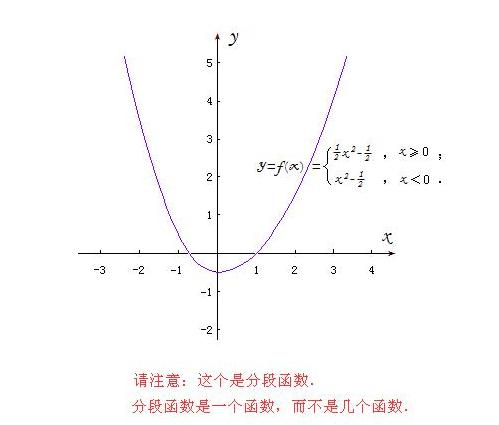2、对于任意一个函数f(x)，自变量当自变量x取两个相反数x、-x时，对应的函数值分别为f(x)、f(-x)： 1）f(x)=f(-x)，f(x)为偶函数； 2）f(x)=-f(-x)，f(x)、f(-x)互为相反数，f(x)为奇函数； 3）f(x)≠f(-x)，但是 f(x)、f(-x)都有意义； 4）f(x)、f(-x)不都有意义，举例说对数函数，如图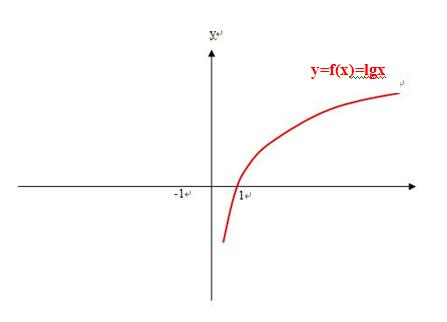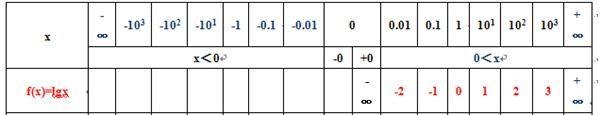3、对于任意一个已知函数f(x)，都有一个函数f(-x)，函数f(-x)的图像与已知函数f(x)的图像以y轴为对称即f(x)=f(-(-x))， 1）如果已知函数f(x)的定义域为x＞0，则与之对应的函数f(-x)的定义域为x＜0，f(x)、f(-x)总有一个没有意义，举例说对数函数，如图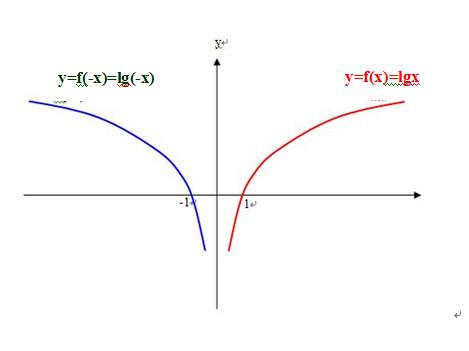2）如果已知函数f(x)为偶函数，那么函数f(-x)的图像与已知函数f(x)的图像以y轴为对称即f(x)=f(-(-x))，且完全重合即f(x)=f(-x)，如图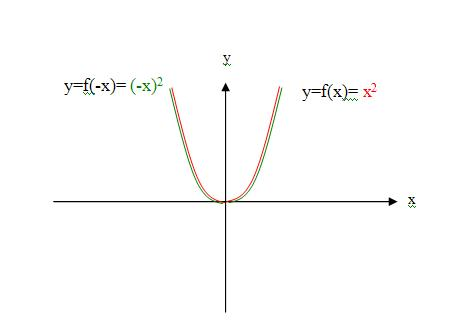3）如果已知函数f(x)为奇函数，那么函数f(-x)的图像与已知函数f(x)的图像以y轴为对称，即f(x)=f(-(-x))，f(-x)也是个奇函数，如图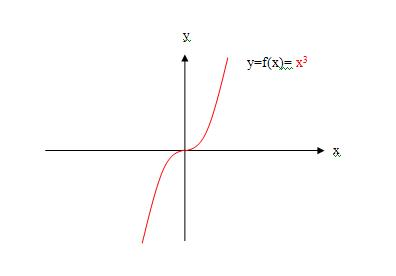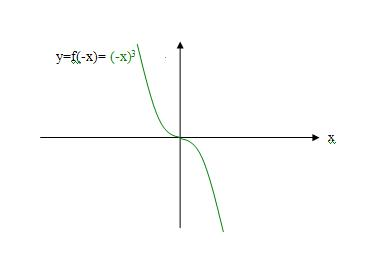4）如果已知函数f(x)为奇函数，那么有函数-f(-x)的图像与已知函数f(x)的图像以原点o为中心对称即-f(x)=-f(-(-x))，且完全重合即f(x)=-f(-x)，-f(-x)与f(x)是两个全等的奇函数，如图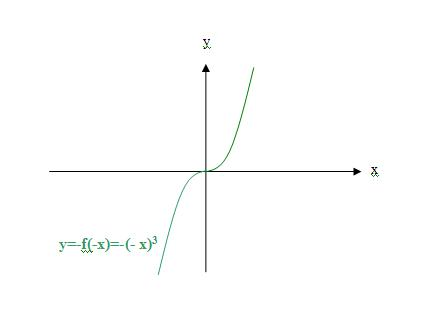5）对于任意一个已知函数f(x)，都有一个函数f(-x)，函数f(-x)的图像与已知函数f(x)的图像以y轴为对称即f(x)=f(-(-x))，如果已知函数f(x)是个分段函数，举例说wanggq 的分段函数f(x)，也有一个分段函数f(-x)，但是f(x)≠f(-x)，f(x)、f(-x)都有意义，如图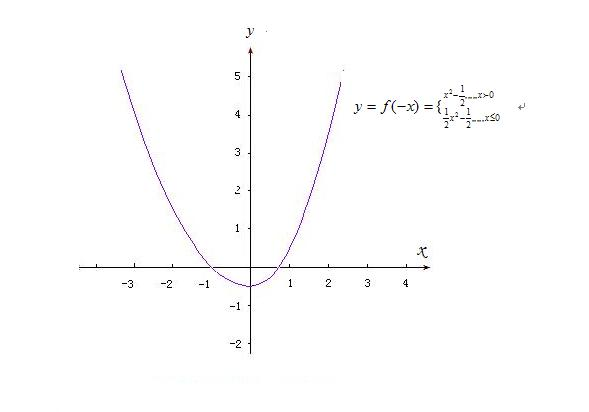4、函数f(x)图像在坐标系中的位置变换包括平移、翻转、旋转，函数图像的形状变化包括伸缩； 1）平移 水平平移：设函数f(x)，则函数f(x+a)的图像为已知函数f(x)的图像向左（a＞0）移动a各单位； 竖直平移:设函数f(x)，则函数f(x)+a的图像为已知函数f(x)的图像向上（a＞0）移动a各单位； 2）翻转 水平翻转：设函数f(x)，则函数f(-x)的图像为已知函数f(x)以y轴翻转180°所得图像； 垂直翻转：设函数f(x)，则函数-f(x)的图像为已知函数f(x)以x轴翻转180°所得图像； 3）旋转 任意角旋转 90°角旋转：设函数y=f(x)，则函数x=f(-y)的图像为已知函数f(x)以原点为转轴逆时针旋转90°所得图像； 180都角旋转：设函数f(x)，则函数-f(-x)的图像为已知函数f(x)以坐标原点为轴旋转180°所得图像； 4）伸缩 水平伸缩：设已知函数f(x)，则函数f(ax)（a＞0）的图像为已知函数f(x)的图像沿水平方向压缩a倍； 竖直伸缩：设已知函数f(x)，则函数af(x)（a＞0）的图像为已知函数f(x)的图像沿竖直方向扩大a倍； 5、下来看看主楼题目属于那种情况： 1）原题“f(x)在0到正无穷大是增函数，f（1）=0，求（f(x)-f(-x)）/x<0时x的范围”； 2）已知函数“f(x)在0到正无穷大是增函数，f（1）=0，”； 3）求解“求（f(x)-f(-x)）/x<0时x的范围”； 4）所以已知函数f(x)的定义域为x＞0，图像在2、4象限，单调递增函数，与x轴有一交点f（1）=0；求解函数为f(-x)、-f(-x)，所以此题目属于已知函数f(x)，求函数f(-x)，属于函数变换中的水平翻转问题； 5）以满足题设条件（在0到正无穷大是增函数，f（1）=0）的对数函数为例，求解过程如下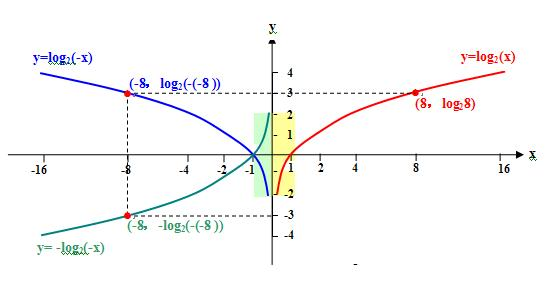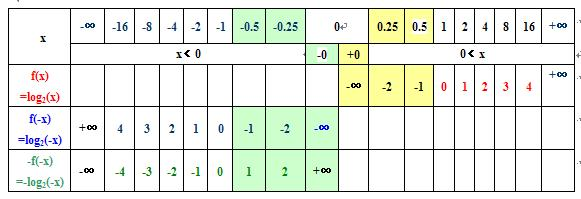-1＜ x＜0      f(x)-f(-x)）/x = -f(-x)/x < 0   如图中绿色部分；             0 ＜x＜1     f(x)-f(-x)）/x = f(x)/x < 0    如图中黄色部分；